Applied and Computational Mathematics
Volume 5, Issue 3, June 2016, Pages: 107-113

Development of a Hybrid Algorithm for Efficiently Solving Mixed Integer-Continuous Optimization Problems

Dianzi Liu

School of Mathematics, Faculty of Science, University of East Anglia, Norwich, UK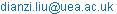Dianzi Liu. Development of a Hybrid Algorithm for Efficiently Solving Mixed Integer-Continuous Optimization Problems. Applied and Computational Mathematics. Vol. 5, No. 3, 2016, pp. 107-113. doi: 10.11648/j.acm.20160503.13

Received: April 20, 2016; Accepted: June 12, 2016; Published: June 15, 2016

Abstract: Problems with mixed integer-continuous design variables are a class of complicated optimization problems that commonly exist in practical engineering design work. In this paper, a hybrid algorithm combining metamodel-based Multipoint Approximation Method (MAM) and Hooke-Jeeves direct search technique is presented to efficiently seek the optimum solutions for mixed integer-continuous optimization problems. First, optimal continuous valuesare obtained by the Sequential Quadratic Programming method (SQP) on the approximated functions in a current trust region. Then, continuous values are rounded to the nearest integer values for discrete variables. Utilizinginteger values as a starting point, the Hooke-Jeeves assisted MAM is applied to search for the discrete optimal solution in the sub-space of discrete variables as well as accordingly update the sub-optimal values for continuous design variables by SQP. The proposed hybrid algorithm is examined by the well established benchmark example and the obtained results demonstratethe superiority of the developed algorithm over GA in terms of computational cost and the quality of solutions.

Keywords: Integer-Continuous Optimization, Multipoint Approximation Method, Metamodel, Direct Search, Hybrid Algorithm

Contents

1. Introduction

Many non-linear optimization problems share the common features: the objective and constraint functions are computationally expensive using numerical methods (e.g., finite element method) or could be impossible to evaluate at some combinations of design variables (e.g., nodal values of displacements, stresses). In order to improve computational efficiency and accuracy of solving suchstructural optimization problems, the Multipoint Approximation Method (MAM) was developed for this purpose [1,2]. The advantage ofthe MAM techniqueis presentedto replace the original optimization problem with a succession of simpler mathematical programming in a scalable trust-region of design space and utilize high-quality explicit approximations to reduce the total number of calls for analyses needed for the complicated optimization problems. Details on the developmentof the MAM technique for various applications are discussed in [3-6]. To enhance the accuracy of metamodels used to approximate the real responses, metamodel assembly approachis implemented in MAM to construct an assembly of multiple surrogates into a single surrogate using linear regression [7,8].

In practical engineering designs, some or all of the design variables have discrete or integer values because the available values for those design variables are limited to a set of standard sizes, for example, the thickness of a steel plate, the diameter of the cross-section in a truss structure, etc. In general, it is only allowed to perform response function evaluations for points that have discrete values of the design variables . New procedures for sampling, metamodel building, their use for solving an optimization problem with continuous and discrete properties within a trust region, and the trust region adaptation strategy have been developed to enhance the MAM with the discrete capability by authors [10,11].

In current research, a discrete form of Hooke-Jeeves direct searchmethod  is implemented within theMAM to search for the optimal solution in the sub-space of the discrete variables only starting from the optimal continuous values obtained by the Sequential Quadratic Programming method (SQP) on the approximated functions in a current trust region. This developed hybrid algorithm combining the metamodel-based MAM and Hooke-Jeeves direct search technique aims at efficiently seeking the best solution of mixed integer-continuous optimization problems. The enhanced discrete capability and efficiency of the hybrid algorithm are examined by the well-established benchmark ten-bar truss example  and the obtained results are compared with the ones by a binary Genetic Algorithm (GA).

2. Multipoint Approximation Method

The MAM is based on the building of mid-range approximations [4-5] and is suitable to solve large-scale optimization problems by producing better quality approximations that are sufficiently accurate in a current trust region and inexpensive in term of computational costs required for their building. These approximation functions have a relatively small number (N+1 where N is number of design variables) of regression coefficients to be determined and the corresponding least squares problem can be solved easily. This feature of such approximations allows applying them to large scale optimization problems with the number of design variables in the order of hundreds.

In general, an optimization problem can be formulated as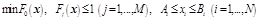(1)

whererefers to the vector of design variables;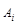and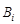are the given lower and upper bounds on the design variable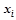;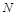is the total number of the design variables;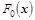is an objective function;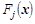is the constraint function andis the total number of the constraint functions.

In order to present the detailed model of a structure by the response functions and reduce the number of calls for the response function evaluations, the MAM replaces the optimization problem by a sequence of approximate optimization problems:(2)

Where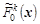an d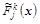are the functions which approximate the functionsandof the initial optimization problem defined in Eq. (1), and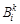are the side constraints of a trust region when searching the solution of the approximate optimization problem,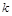is the iteration number.

The selection of the approximate response functions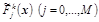is such that their evaluation is inexpensive as compared to the evaluation of the response functions, although they are not necessarily explicit functions of the design variables. The approximate response functions are intended to be adequate in a current trust region. This is achieved by appropriate planning of numerical experiments and use of the trust region defined by the side constraints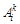and.

3. Design of Experiments and Trust Region Strategy

Metamodels built in MAM are used to approximate the objective and constraint functions in a trust region at each iteration of the optimization process. The quality of the metamodels strongly depends on an appropriate choice of the Design of Experiments (DoE) type and sampling size, since DoE could have a direct impact on the efficacy of metamodels. In the MAM technique, DoE should generate sampling points taking into account the discrete nature of some of (or all) the design variables. This ensures that the calls for the response function evaluations are only made for the points that have discrete values of the design variables. The added points are checked for calculability of the response function and, if the check fails (i.e. the simulation software did not return the response function values), a new set of points is generated until a required number of sampling points (all passing the check) are obtained.A strategy to improve the quality of sampling points - constraint assisted DoE rather than random DoE - is applied in this paper. The assisted DoE generates the sample points with a constraint on the minimal distance between the points using the following expression: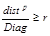(3)

where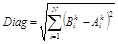,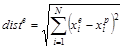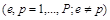,

Diag is a characteristic size of the trust region,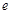is a number of a new sampling point,  is a number of a previously generated point,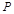is the total number of sampling points in the trust region,is the total number of the design variables,andare the side constraints of a trust region when searching the solution of the approximate optimization problem, k is the iteration number. The parameter r is initially set to 0.9 and if the assessment on Eq. (3) fails after a prescribed number of random generations for a new point, the value r will be iteratively reduced by multiplying a positive constant (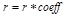,) until the Eq. (3) is satisfied. Imposing such a mechanism on the selection of points, the uniformdistributionof sampling points across the space is guaranteed. As an example, a 30-point pattern with a constraint imposedon the minimal distance for design variables only taking discrete values is shown in Fig. 1.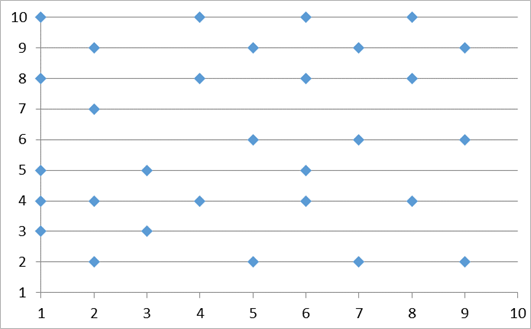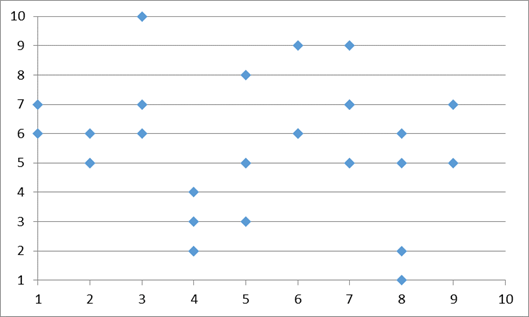Fig. 1. 30-point patterns with (top) and without (bottom) a constraint imposed on the minimal distance when design variables only take discrete values.

The aim of the trust region strategy [14-16] developed in the MAM technique is to controlthe quality of a metamodel in terms of the quality of fit. When the approximation gets better, the trust region will be further reduced. The track of the trust regions also indicates a path of the direction from the initial starting point to the optimum over the entire searching domain. At each MAM iteration, a new trust region must be defined, i.e. its new size and its location have to be specified. It should be noted that if the sub-optimum point does not pass the check for calculability of the response functions, the trust region is reduced and the approximated problem is solved again. The only essential assumption here is that all functions of the optimization problem exist at the starting point. Note that for the discrete variables there is a restriction on the trust region size reduction, i.e. the corresponding size of the trust region has to contain at least three levels of a discrete variable.

4. Using an Assembly Technique to Build Approximations

Based on the research on multiple metamodels [6-8], a two-step regression procedure is applied to build the metamodel represented by the assembly of different approximations. This was introduced in the form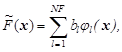(4)

wheremeans different approximate models, which are assembled into one metamodel; NF is the number of regressors in the approximate models {};is the corresponding regression coefficient and reflects the accuracy of the individual surrogates on a set of validation points.

In order to build the expression in Eq. (4), the optimization of the minimization of the expression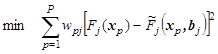(5)

is formulated to determine the regression coefficients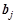. This weighted least squares problem leads to solving a linear system of NF equations with NF unknowns. Here the regression coefficientsshould not be considered as weight factors, e.g. could be either positive or negative. The parameters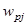refer to the weights that reflect the inequality of data obtained at different sampling points [4, 5].

The functions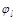in (4) are determined in the similar manner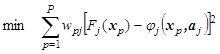(6)

where the minimization is carried out with respect to the tuning parameters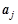,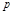means the number of points used in a specified DoE (see Section 3). Note that the tuning parametersin Eq. (6) should be determined before the procedure (5) has been applied.

The evaluation of the regressors  is based on the data from the sampling points currently located in the trust region. In the mid-range approximation framework, inexpensive approximate models for objective and constraint functions are built using minimum required number of sampling points. The simplest case is a linear function and more complex ones are intrinsically linear functions  that have been successfully used for a variety of design optimization problems. Intrinsically linear functions are nonlinear but they can be led to linear ones by simple transformations. In this paper, the intrinsically linear functions considered in the model bank {} are: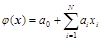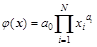(7)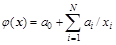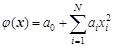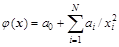5. Hooke-Jeeves Search Technique for Discrete Optimization

The strategy for discrete optimization performed in the trust region can be described as follows: In each iteration of MAM, a continuous solution is initially sought in a current trust region using the SQP method applied to the approximated optimization problem formulated in Eq.(2). The discrete values are then obtained by rounding the continuous variables to the nearest discrete ones. Fixing these rounded values for discrete design variables, a continuous optimization is performed again with the reduced number of design variables, which include only continuous variables. Then, a discrete form of Hooke-Jeeves direct search techniqueis implemented within the MAM to search for the optimal solution for mixed integer-continuous optimization problems at the current iteration. This temporary optimal solution becomes a starting guess for the next iteration of the optimization process until the final optimum is found.

The Hooke-Jeeves direct searchtechnique [12,18,19] examines points near the current point (having the rounded values for discrete design variables) by perturbing design variables - one variable at a time - until an improved point is found. There is a similarity between Hooke-Jeeves technique and the coordinate search algorithm previously suggested in  in terms of the determination of the optimal search path for the solution. The Hooke-Jeeves search technique begins with the starting point, as well as 2Nd coordinate points, where Ndis the number of discrete design variables. The ith pair of coordinate points differs from the starting point only in the ith coordinate. The next test point will be places by a perturbation Δi (positive or negative) along the ith coordinate. The size of Δi is determined by the spacing of the ith discrete design variable. The point with the lowest objective function value (that is penalized in case of violated constraints) along ith coordinate will be saved and then, a further perturbation Δi along the preceding direction is taken into account as well as optimal values for the continuous variables are updated accordingly. This search process will terminate when a worse objective function value is identified. Finally, the latest saved optimum is assigned to the ith discrete design variable. This search strategy are repetitively applied for the next (i+1) th coordinate search until all the coordinates representing discrete design variables are considered.

As direct search algorithms  are known as unconstrained optimization techniques, an exterior penalty function is used to accommodate the constraints by penalizing unfeasible solutions as follows:(8)

where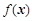is the objective function penalized is case any of the constraints is violated,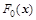is the objective function,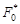is the initial value of objective function at the starting point,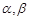are the penalty parameters, here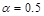and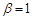are suggested,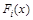is the ith constraint function,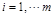,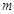is the total number of constraints.

Every time when the discrete variables are modified by the discrete optimizer, it is necessary to make an adjustment of the remaining, continuous design variables so that the optimal values for both discrete and continuous design variables are achieved at the current iteration. This is carried out by the use of SQP in the space of continuous variables while the discrete variables are assigned by the current discrete optimum. Therefore, the overall local optimization process that is carried out on the approximated response functions, is performed in two levels. The outer optimization loop deals with the discrete variables only and is based on the Hooke-Jeeves direct search technique, and the inner optimization loop adjusts the continuous design variables using SQP.

6. Ten-Bar Truss Structure

6.1. Example 1

The developed hybrid algorithm is tested on the well known ten-bar truss benchmark problem  shown in Fig. 2 that has been used by many authors. The minimum weight design is obtained by changing the cross-sectional areas (from 0.1 in2 to 12.7 in2 with the increment of 0.2) of the truss members subject to stress constraints and minimum gage constraints of 0.1in2. The allowable stress in each truss member is the same in tension and compression and is set to 25 кsi for all members except member 9 for which it is 75 кsi. The density of the truss material is 0.1 lb/in3, the member size L=360 in, the loads P1 = P2 = 100 Kips and P3 = 0.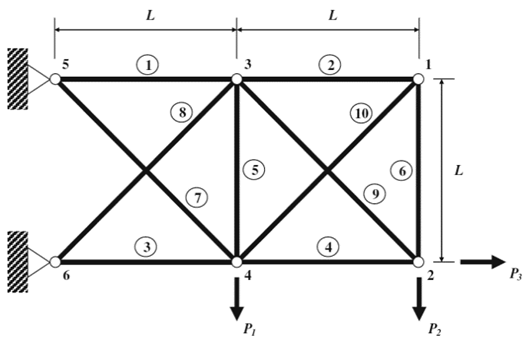Fig. 2. Ten-bar truss structure.

The results for weight optimization of the ten-bar truss structure are presented in Table 1 and information on the constraint values for the optimal design is listed in Table 2. The number of sampling points used in each iteration is 15. In the discrete optimization of ten-bar truss, the obtained results were compared to the results obtained by a binary genetic algorithm (GA). Since a GA is naturally suitable to the discrete optimization and it is also a global search algorithm, a better result from GA could be expected. On the contrary, optimization by the hybridalgorithm(MAM with the implementation of Hooke-Jeeves search technique) has achieved the lighter weight (1546.0) than the result (1560.4) by GA, that is the best result out of five separate GA runs. As expected, a large number of iterations and response analyses in the optimization by binary genetic algorithm are required. The number of iterations (11) for the hybridalgorithm is reduced to 20% of the total number required by GA (57). Hence, the computational efficiency of the hybrid algorithm is higher than GA. Both of optimal designs are feasible solutions with no violation of constraints (see Table 2).

Table 1. Optimal results of discrete optimizations.

 Design variables Discrete variables, in2 (GA, ) Discrete variables, in2 (MAM with Hooke-Jeeves technique) x1 7.7 7.7 x2 0.5 0.3 x3 8.5 8.3 x4 3.7 3.9 x5 0.1 0.1 x6 0.5 0.3 x7 6.3 6.1 x8 5.1 5.5 x9 3.7 3.7 x10 0.7 0.5 Obj. (lb) 1560.4 1546.0 No. of iterations 57 11 No. of response analyses 7157 166

Table 2. Constraints results of discrete optimizations.

 Constraints (ksi) Discrete variables, in2 (GA, ) Discrete variables, in2 (MAM with Hooke-Jeeves technique) G1 24.5 25.0 G2 22.6 25.0 G3 24.8 25.0 G4 24.0 23.7 G5 1.0 2.0 G6 22.6 25.0 G7 25.0 25.0 G8 24.6 23.8 G9 33.9 35.4 G10 22.8 21.2

6.2. Example 2

In this example all the design parameters are the same as the ones in Example 1, but the allowable stress for the member 9 is set to 25 кsi. The optimal results and constraint values for weight optimization of this ten-bar truss structure are presented in Table 3 and 4, respectively. Results from the discrete optimizations of this ten-bar truss structure using the hybrid algorithm have been compared with the ones in [20-22] in Table 3. Optimization by the hybrid algorithm has achieved the best result (the lightest weight, 1610.1) and it is much better than the discrete results by GAs. The number of response analyses for the hybrid algorithm (406) has been reduced by at least an order magnitude as compared to the results from other published paper in Table 3. It is concluded that the computational efficiency of the Hooke-Jeeves search technique implemented in MAM for the discrete optimization are quite higher than GA. All the optimal designs obtained by discrete optimizations are feasible solutions with no violation of constraints (see Table 4).

Table 3. Optimal results of discrete optimizations.

 Design variables Discrete variables, in2 (GA, ) GA, in2  Discrete variables, in2  Discrete variables, in2 (MAM with Hooke-Jeeves technique) x1 7.7 N/A 8.1 7.9 x2 0.5 0.1 0.1 x3 8.5 8.1 8.1 x4 3.7 4.1 4.1 x5 0.1 0.1 0.1 x6 0.5 0.1 0.1 x7 6.3 5.9 5.7 x8 5.1 5.7 5.7 x9 3.7 5.7 5.7 x10 0.7 0.1 0.1 Obj. (lb) 1627.5 1635 1627.5 1610.1 No. of iterations 57 N/A 120 27 No. of response analyses 7206 17906 5190 406

Table 4. Constraints results of discrete optimizations.

 Constraints (ksi) Discrete variables, in2 (GA, ) Discrete variables, in2  Discrete variables, in2 (MAM with Hooke-Jeeves technique) G1 24.5 24.5 25.0 G2 15.1 15.1 14.9 G3 24.9 24.8 24.9 G4 24.0 24.0 24.0 G5 0.1 0.14 1.1 G6 15.1 15.1 14.9 G7 24.3 24.3 25.0 G8 24.4 24.4 24.5 G9 24.4 24.4 24.4 G10 21.3 21.3 21.0

6.3. Example 3

A mixed integer-continuous design problem has been solved in this example. The optimization formulation has been defined as:

Objective: Minimize Weight

Constraint: The allowable stress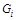,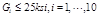except for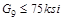;

Design Variables: Cross-sectional areas are changing from 0.1 in2 to 12.7 in2; Continuous cross-sectional areas for truss member 1 to 6 (horizontal and vertical members); Discrete cross-sectional areas for truss member 7 to 10 (diagonal members) with the increment of 0.2.

Table 5. Optimal results of the mixed integer-continuous optimizations (discrete design variables shown in bold).

 Design variables Discrete variables, in2 (GA, ) Discrete variables, in2 (MAM with Hooke-Jeeves technique) x1 7.75 7.81 x2 0.21 0.21 x3 8.25 8.19 x4 3.80 3.83 x5 0.22 0.10 x6 0.27 0.22 x7 (discrete) 6.7 5.9 x8 (discrete) 5.3 5.5 x9 (discrete) 4.3 3.5 x10 (discrete) 0.3 0.3 Obj. (lb) 1583.3 1506.7 No. of iterations 114 32 No. of response analyses 14901 481

Table 6. Constraints results of the mixed integer-continuous optimizations (discrete design constraints shown in bold).

 Constraints (ksi) Discrete variables, in2 (GA, ) Discrete variables, in2 (MAM with Hooke-Jeeves technique) G1 25.0 24.9 G2 24.6 24.9 G3 25.0 25.0 G4 25.0 24.8 G5 5.1 1.7 G6 19.0 24.6 G7 22.4 25.0 G8 25.0 24.4 G9 31.2 38.3 G10 24.4 25.0

In Table 5, the optimal designs of the ten-bar truss structure by GA and the developed hybrid algorithmare presented. Since the optimal design obtained by the hybrid algorithm (1506.7) has reduced the weight by 5% as compared to the one by GA (1583.3), more constraints have been activated or become critical in the converged solution shown in Table 6. As expected, the number of iterations and response analyses in the mixed integer-continuous optimization by the hybrid algorithm are significantly reduced. The number of response analyses for GA (14901) has been reduced by 96.8% as compared to the result by the hybrid algorithm (481), while the lighter weight is also achieved by the latter. In terms of the computational efficiency, the hybrid algorithm for the optimization of this truss structure is much higher than GA. MAM, enhanced by the discrete optimization capability, outperforms a GA not only in the efficiency but also in the quality of the obtained solution. In Table 6, optimal solutions are feasible and no constraint violation happens in these two discrete optimizations.

7. Conclusions

The proposed hybrid algorithm in this paper, combining the metamodel-based MAM and the Hooke-Jeeves search technique, has successfully solved the benchmark problem of a ten-bar truss structure. It indicates that MAM with the implementation of the Hooke-Jeeves search technique is a robust method and it outperforms a GA not only in the efficiency but also in the quality of the obtained solutions. Since it requires much fewer response analyses and less computational cost, the developed hybrid algorithm as an ideal tool can be usedto solve the complicated industrial projects where it is essential to consider the mixed integer-continuous problem formulations in the optimization design process.

References

1. R. T. Haftka, J. A Nachlas., L. A. Watson, T. Rizzo, R. Desai, "Two point constraint approximations in structural optimization," Comput. Methods Appl. Mech. Engrg., vol. 60, pp. 289-301, 1987.
2. V. V. Toropov, "Simulation approach to structural optimization," Struct. Multidisc. Optim., vol. 1, pp. 37-46, 1989.
3. G. M. Fadel, M. F. Riley, J. M. Barthelemy, "Two point exponential approximation method for structural optimization," Struct. Multidisc. Optim., vol. 2, pp. 117-124, 1990.
4. L. P. Wang, R. V. Grandhi, "Improved two-point function approximations for design optimization," AIAA J., vol. 33, pp. 1720-1727, 1995.
5. F. van Keulen, V. V. Toropov, "New development in structural optimization using adaptive mesh refinement and multipoint approximations," Eng. Opt., vol. 29, pp. 217-234, 1997.
6. A. Polynkin, V. V. Toropov, "Mid-range metamodel assembly building based on linear regression for large scale optimization problems," Struct. Multidisc. Optim., vol. 45, pp. 515-527, 2012.
7. F. A. C. Viana, R. T. Haftka, "Using multiple surrogates for metamodeling," Proceedings of 7th ASMO-UK/ISSMO International Conference on Engineering Design Optimization, pp. 132-137, Bath, UK, July 7-8, 2008.
8. E. Acar, M. Rais-Rohani, "Ensemble of metamodels with optimized weight factors," Struct. Multidisc. Optim., vol. 37, pp. 279-294, 2009.
9. V. O. Balabanov, G. Venter, "Response surface optimization with discrete variables," Proc. 45th AIAA/ASME/ASCE/AHS/ASC Structures, Structural Dynamics and Materials Conference, Palm Springs, CA, AIAA 2004–1872, April 19-22, 2004.
10. D. Liu, V. V. Toropov, "Multipoint approximation method for design optimization with discrete variables," 14th AIAA/ISSMO Multidisciplinary Analysis and Optimization (MAO) Conference, Indianapolis, IN, 17–19 September, 2012.
11. D. Liu, V. V. Toropov, "Implementation of discrete capability into the enhanced multipoint approximation method for solving mixed integer-continuous optimization problems," International Journal for Computational Methods in Engineering Science & Mechanics, 17. pp. 22-35, 2016
12. T. G. Kolda, R. M. Lewis, V. Torczon, "Optimization by direct search: new perspectives on some classical and modern methods," SIAM Rev., vol. 45, pp. 385-482, 2003.
13. R. T. Haftka, Z. Gürdal, Elements of Structural Optimization, 3rd ed., Kluwer Academic Publishers, 1992.
14. G. H. Cheng, A. Younis, K. H. Hajikolaei, G. G. Wang, "Trust region based mode pursuing sampling method for global optimization of high dimensional design problems," Journal of Mechanical Design 137 (2): 021407, 2015.
15. Y. Yuan, "Recent advances in trust region algorithms," Mathematical Programming, 151 (1), pp 249-281, 2015.
16. A. Kamandi, K. Amini, M. Ahookhosh, "An improved adaptive trust-region algorithm," Optimization Letters, pp 1-15, 2016, DOI: 10.1007/s11590-016-1018-4
17. G. E. P. Box, N. R. Draper, "Empirical model-building and response surfaces," New York: John Wiley and Sons, 1987.
18. Y. S. Ong, P. Nair, A. Keane, "Evolutionary optimization of computationally expensive problems via surrogate modeling," AIAA Journal, 41 (4), pp. 687- 6 96, 2003
19. X. D. T. Garcia, F. Neri, G. L. Cascella, N. Salvatore, "A surrogate assisted hooke-jeeves algorithm to optimize the control system of a pmsm drive," 2006 IEEE International Symposium on Industrial Electronics, pp 347 - 352 DOI: 10.1109/ISIE.2006.295618, 2006
20. HyperStudy V 12.0, Altair Engineering Inc, USA, 2012.
21. P. Hajela, J. Yoo, "Constraint handling in genetic search using expression strategies," AIAA J., vol. 134, pp. 2414-2420, 1996.
22. S. Y. Mahfouz, "Design Optimization of Structural Steelwork," PhD thesis, University of Bradford, Bradford, UK, 1999.

 Contents 1. 2. 3. 4. 5. 6. 6.1. 6.2. 6.3. 7.
Article ToolsAbstractPDF(606K)